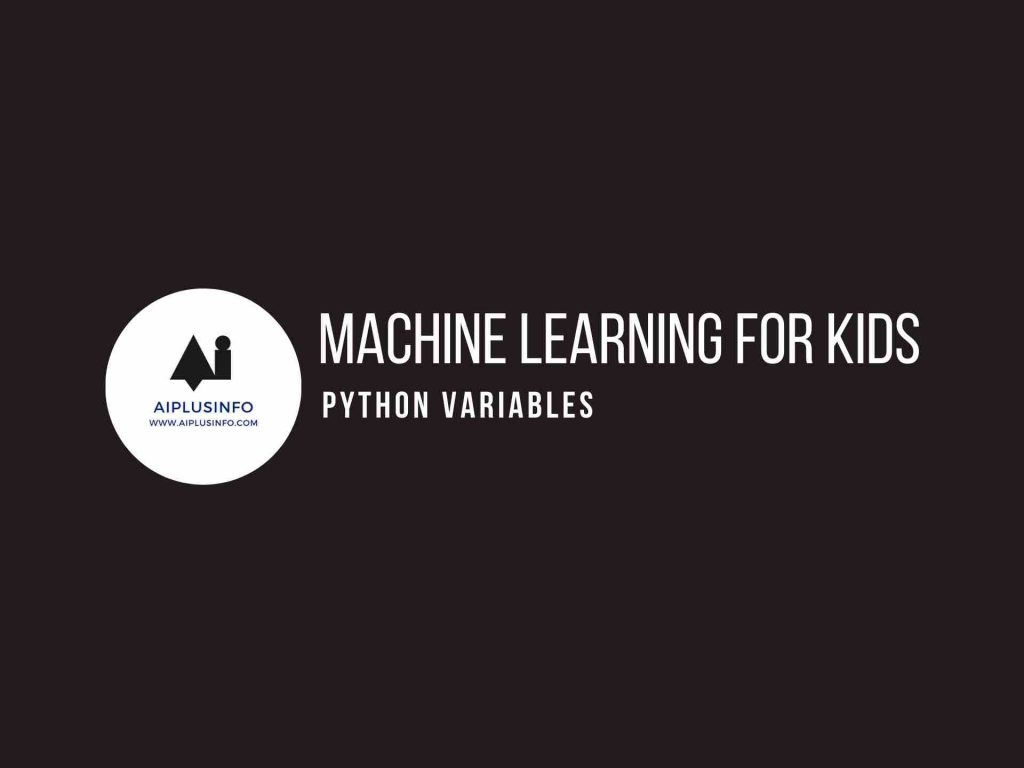# Machine Learning For Kids: Python Variables## Introduction

Machine learning for kids: Welcome to this tutorial on Python Variables! Variables are the backbone of any programming language, serving as containers where we store our data. They’re like little boxes where we can put things for use later.

## Who Is This For?

This tutorial is designed for students in grades 6 through 10. Whether you’re completely new to Python or have dabbled a bit in programming, this tutorial will help you understand the fundamental concept of Python variables.

## What Will We Learn?

In this journey of “Machine Learning for Kids” lets learn the basics of python which is the foundation of machine learning. In this tutorial, we’ll dive into what variables in Python are, how to create them, and how we can use them. We will discuss the different types of data we can store in variables and illustrate this with easy-to-understand examples.

Also Read: How Long Does It Take To Learn Python

## Understanding Python Variables

In Python, a variable is created when you assign a value to it. For example, `x = 5` creates a variable named `x` and stores the number `5` in it. Python variables don’t require explicit declaration to reserve memory space. The declaration happens automatically when you assign a value to a variable.

You can think of variables as labels for containers that hold information or data. The data stored in a variable, known as its value, can be of various types like numbers, text (known as strings in Python), lists, and more.

Let’s illustrate this with some code:

``````# Creating variables
name = "John"
age = 12
fruits = ["apples", "bananas", "cherries"]

# Printing variables
print(name)
print(age)
print(fruits)``````

Here’s what’s happening in the code:

`name = "John"`: Here, we’re creating a variable called `name` and storing the string “John” in it.

`age = 12`: This creates a variable called `age` and stores the number `12` in it.

`fruits = ["apples", "bananas", "cherries"]`: This creates a variable called `fruits` and stores a list of strings in it.

`print` is a function that displays the value of a variable to the console. When we print our variables, we see the values that are stored in them:

``````John
12
['apples', 'bananas', 'cherries']``````

As you can see, Python variables are flexible and allow us to store various types of data, including numbers, strings, and lists. In subsequent tutorials, we’ll explore more about different data types and how we can operate on them in Python. Happy coding!

## NEXT TUTORIALS

Tutorial 1 – Installing Python
Tutorial 2 – Your First Program in Python
Tutorial 3 – Python Variables
Tutorial 4 – Python Data Types
Tutorial 5 – Python Conditionals
Tutorial 6 – Python Loops
Tutorial 7 – Python Functions
Tutorial 8 – Advanced Python Functions
Tutorial 9 – Starter Machine Learning Python Program
Tutorial 10 – Your First Machine Learning Program!

Explore more with this book.

Python for Kids, 2nd Edition: A Playful Introduction to Programming
\$22.99
We earn a commission if you make a purchase, at no additional cost to you.
09/18/2023 12:47 am GMT

## References

Briggs, Jason R. Python for Kids: A Playful Introduction To Programming. No Starch Press, 2012.